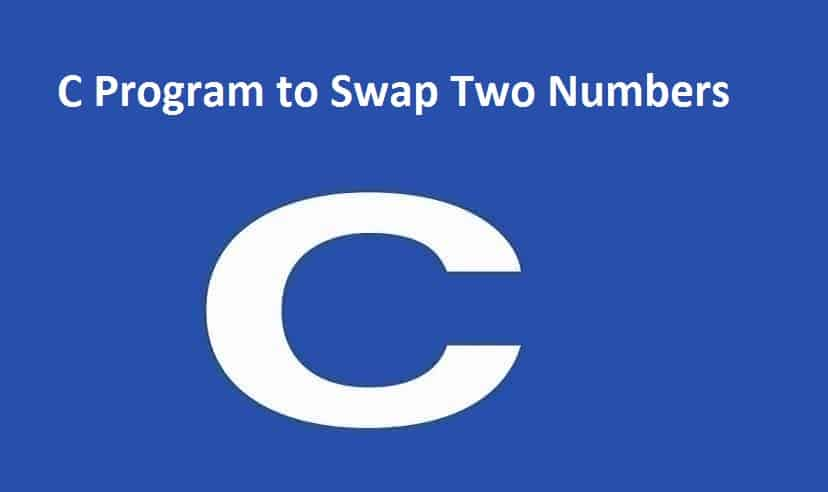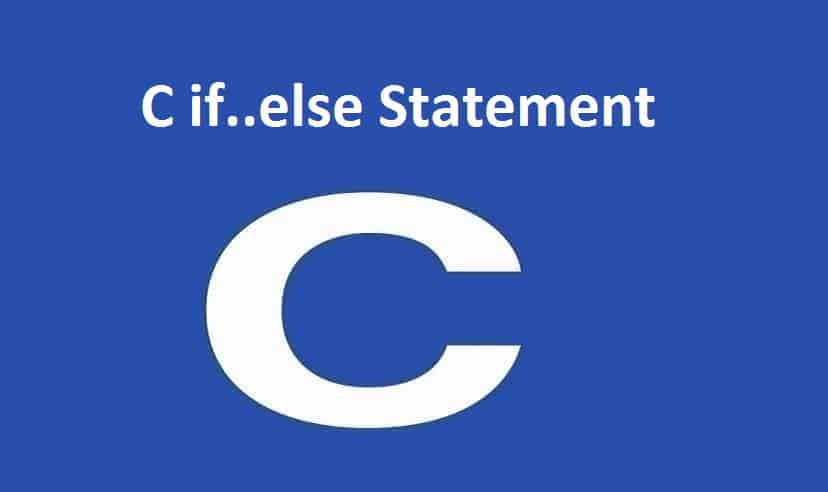# C Program to Swap Two Numbers## C Program to Swap Two Numbers

In this example, you will learn to swap two numbers in C programming using two unique strategies.

To understand this example, you should have knowledge of the following C programming topics:

C Data Types

C Programming Operators

C Input-Output (I/O)

## Swap Numbers Using Temporary Variable

```#include<stdio.h>
int main() {
double first, second, temp;
printf("Enter first number: ");
scanf("%lf", &first);
printf("Enter second number: ");
scanf("%lf", &second);

// Value of first is assigned to temp
temp = first;

// Value of second is assigned to first
first = second;

// Value of temp (initial value of first) is assigned to second
second = temp;

printf("\nAfter swapping, firstNumber = %.2lf\n", first);
printf("After swapping, secondNumber = %.2lf", second);
return 0;
}```

Output

```Enter first number: 1.20
Enter second number: 2.45

After swapping, firstNumber = 2.45
After swapping, secondNumber = 1.20```

In the above program, the temp variable is assigned the value of the first variable.

Then, the value of the first variable is appointed to the second variable.

Finally, the temp (which holds the initial value of first) is assigned to second. This completes the swapping process.

## Swap Numbers Without Using Temporary Variables

```#include <stdio.h>
int main() {
double a, b;
printf("Enter a: ");
scanf("%lf", &a);
printf("Enter b: ");
scanf("%lf", &b);

// Swapping

// a = (initial_a - initial_b)
a = a - b;

// b = (initial_a - initial_b) + initial_b = initial_a
b = a + b;

// a = initial_a - (initial_a - initial_b) = initial_b
a = b - a;

printf("After swapping, a = %.2lf\n", a);
printf("After swapping, b = %.2lf", b);
return 0;
}```

Output

```Enter a: 10.25
Enter b: -12.5
After swapping, a = -12.50
After swapping, b = 10.25```

Please feel free to give your comment if you face any difficulty here.

For more Articles click on the below link.### C Program to Find the Size of int, float, double and char### C if..else Statement